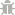BETA
Light Mode
Rate us!# Probability Calculator

Single Probability Calculator
Number of possible outcomes
Number of events occured
Multiple Event Probability Calculator
Number of possible outcomes
Number of event occurs in A
Number of event occurs in B
Note: A & B are mutually exclusive events

An online probability calculator provides you the opportunity to find the probability of an event based on probabilities of other events. You can use our free online tool from any part of the world without paying anything.

With an online tool, you can examine the associations between two discrete events within no time. You don’t need to make any calculations by yourself anymore, as an online tool will provide you with accurate results in a few seconds. Moreover, there is no need to waste your energy and time on calculating complex values to find the probability. Our calculator allows you to get rid of the conventional methods of calculating probability and enables you to find precise results quickly.

## How to Use Online Probability Calculator?

The method of using our Calculator is easy and straightforward. There are no hard and fast rules to follow for finding the probability with this online Conditional Probability Calculator.

You can use this tool in two ways. Whether you want to find the probability for a single event or multiple events, this online Experimental Probability Calculator is the most suitable option for you.

## Probability Calculator Single Event

• Click on the “Single” button for finding a single event probability.
• Enter the number of possible outcomes in our single event probability calculator.
• Once you entered the possible outcomes, enter the number of events that occurred.
• That’s all! You will get the probability of an event that occurs and an event that doesn’t occur within a few seconds.

## Probability Calculator Multiple Events

If you want to find the probability of multiple events, then our tool is the best option that you could find over the web. For finding the probability of multiple events, you have to:

• Enter the number of possible outcomes directly in the given box.
• Insert the number of events occurs in set A, and set B in the designated boxes.
• As a result, you will get a comprehensive report including P(A), P(B), P (A∩B), P(A∪B), P(A’), P(B’), and P(A|B).

## What Is Probability?

Probability measures the chances of an event to occur, that either it will happen or not. You may have an idea that probability is the branch of mathematics that is based on the study of random events. It is already understood that the outcome of a random occurrence may not be predicted until it occurs, but probability enables us to find the chances of this occurrence. Probability works on the basis of logical factors and allows us to find if an event will have a high or low chance of occurring. You may also get to know that the event will be dependent or independent from the previous occurred events through probability.

## How to Calculate Probability?

To calculate probability, you need to separate a problem into individual probabilities and then multiply the chances of an event’s occurrence with one another. You can find the probability by following the discussed simple steps.

• Analyze the probability of an event that has at least one possible outcome. For example, conclude the possible outcomes of tossing a coin is that either you will get head or you won’t get heads.
• Now, identify the total number of outcomes for the event measured in the last step. In the example of tossing a coin, the total number of results is 2, i.e., head and tail.
• Lastly, divide the chances of an event’s occurrence with the total number of results. Continuing with the ongoing example, the possible number of getting heads after flipping a coin is 1, and the total number of results is 2. So, the probability of getting heads can be calculated as 1/2, =0.5

## Find The Probability of A Union B (AUB)?

In simple words, the union of set A and set B is the collection of two all the values in both the sets. The union of A and B can be denoted by A∪B. To understand the working of the union, let’s take an example.

A = {2, 3, 5, 6, 7}

B = {2, 3, 9}

The A∪B for this example would be A∪B = {2, 3, 5, 6, 7, 9}

However, the easiest way to avoid any manual calculation is the use of our Statistics Probability Calculator.

## Find The Probability of A Intersection B (A∩B)?

In the intersection of A and B, we will find out all the similar values that are existed in both the sets, set A, and set B. The intersection of set A, and B, will be denoted by (A∩B).

To understand the intersection, the example could be:

A = {4, 6, 3, 8, 9}

B = {5, 6, 3}

The values that exist in both sets are 6 and 3. So, A∩B = {6,3}

## Find the Conditional Probability of A and B: P (A | B)

The conditional probability can be measured as the computation of the event A probability, given that event B has occurred. The following probability formula will help you to calculate the conditional probability of A and B.

P(A|B) = P(A∩B) P(B).